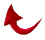IN THE NAME OF GOD MOST GRACIOUS MOST MERCIFUL

JOURNAL OF SUBMISSION

Volume 1 No. 11.5, January-February 1994

Editor: Ali R. Fazely, Ph.D.

Associate Editors: Saied Andalib and Milan Sulc

P.O. Box 80333

Baton rouge, Louisiana 70808

Base 8 or Octal Number System

Once again, God has blessed the believers and the subscribers to the Journal of Submission with reassurance from Him that they are on the right path.  I am sending this “extra” issue to inform you of a most sophisticated number system that God has shown us on Friday, after our Jum’a prayer here in Baton Rouge.  After I went to my office and was learning how to use my new laser printer which I use for my research, I wondered if I could print the calculator on the laser printer.  This was the start of another awesome miracle from The Most Gracious.  My calculator has a mode function which I have never used before.  When I clicked on this function, I noted that it has three functions, or three number systems, namely decimal (base ten), hexadecimal (base 16), and octal (base 8).  Immediately, I tried 19 and converted it into octal, it is 23.  I could not stop, and I was praising God as I was discovering new marvels.  Let me explain how.  The following table shows some key Quranic numbers (assumed to be in decimal system) written in Octal.

 Decimal to Octal Conversion Table Decimal System Octal System 8 10 19 23 23 27 30 36 38 46 55 67 67 103 76 114 89 131 337 521

Remember 69:17, “On that day His dominion will encompass 8”.  We also know that the 8th prime is 19.  Now let us look at the above table.  Eight in decimal is 10 in octal, note that the 10th initialed sura is 19.  Nineteen is 23 in octal.  Remember that 19 is the value of “ONE” and 23 is the value of “ALONE”, regardless of grammatik or sequential value of the Arabic alphabet.  23 is 27 in octal, and remember 27 = 19 + 8.  Note that these numbers are not readily transparent if they were meant to be in decimal or octal, unless they contain 8 or 9 explicitly.  30 which is the 19th composite is 36 written in octal and 36 is the 19th initialed sura.  38 which is the sequential value of the word Muhammad (also the number of verses in sura Muhammad) is 46 written in octal which is the sequential value of the word Rashad.  Sura 55 is the Most Gracious and 55 written in octal is 67 which is the 19th prime.  67 written in octal is 103 which is the 27th prime and 27 = 19 + 8.

The first five revelations of the Quran revealed to prophet Muhammad contained 76 Arabic letters and 76 written in octal is 114 and let us praise God for His infinite grace.  We know that both chapters 10 and 11 (10 and 11 in octal are 8 and 9 in decimal) in the Quran contains the same number of initials A + L + R and it is equal to 2489 = 19 x 131.  From the above table 89 written in octal is 131 and 24 is the index of prime number 89.  Note that 24 written in octal is 30 and 30 is the 19th composite and 89 has 30 verses.  How is that for Ultimate Mathematics?  Note that there are no mathematics know to man or jinn to tackle the mathematics of the Quran.

Ultimate Mathematics

God will not leave the disbelievers alone,  One of the functions of mathematics of the Quran is to disturb the disbelievers.  Note the last number in the above table namely 337 and 521.  We know that 521 is the coefficient of 19 in the first initialed sura, sura 2.  One of the functions of 521 is to predict the last initial sura 68.  Guess what? 337 is the 68th prime number.  Remember that the521st TPC is 33768.  There is no way we can connect these numbers to each other mathematically.  The only way to explain this is that numbers have submitted to God and so should the humans.Return to Table of Contents